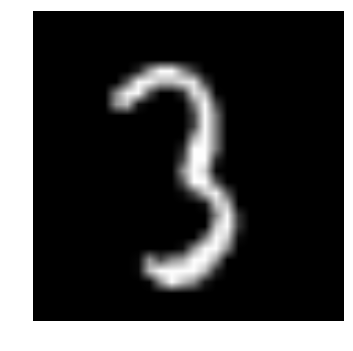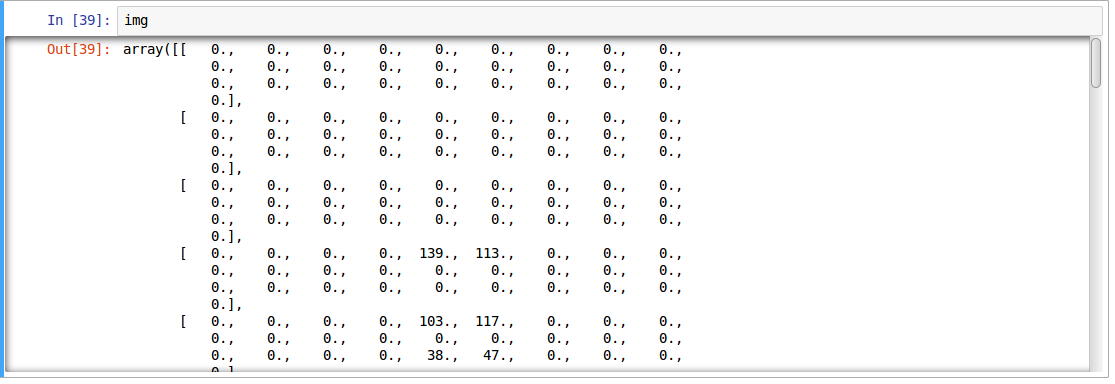# 曹佳润

Jerry Cao

Welcome to my homepage. The English version is coming very soon. Please stay tuned.

## 介绍

一直关注 `数据科学``机器学习` 的同学，一定会经常看到或听到关于 `深度学习``神经网络` 相关信息。如果你对 `深度学习` 感兴趣，但却还没有实际动手操作过，你可以从这里得到实践。

在本文中，我将介绍 `TensorFlow` , 帮你了解 `神经网络` 的实际作用，并使用 `TensorFlow` 来解决现实生活中的问题。 读这篇文章前，需要知道 `神经网络` 的基础知识和一些熟悉编程理念，文章中的代码是使用 `Pyhton` 编写的，所以还需要了解一些 `Python` 的基本语法，才能更有利对于文章的理解。### 什么时候用神经网络？

`神经网络` 已经在相当一段时间成为机器学习中的焦点。 对于 `神经网络``深度学习` 上这里有更详细的解释 点击阅读 。 其 “更深” 的功能在许多领域都有取得巨大的突破，如图像识别，语音和自然语言处理等。

主要的问题在于如何用好 `神经网络` ？现在，每天都会有许多新发现，这个领域就像一个金矿，为了成为这个 “淘金热” 的一部分，必须记住几件事：

• 首先，`神经网络` 需要有明确和翔实的数据（主要是大数据）训练， 试着想象 `神经网络` 作为一个孩子，它一开始会观察它父母走路，然后它试图自己走，每一步就像学习执行一个特定的任务。 它可能会失败几次，但经过几次失败的尝试，它将会如何走路。所以需要为孩子提供更多的机会，如果不让它走，它可能永远不会学习如何走路。

• 一些人会利用 `神经网络` 解决复杂的问题，如图像处理，  `神经网络` 属于一类代表学习的算法，这些算法可以把复杂的问题分解为简单的形式，使他们成为可以理解的（或 “可表示”），就像吞咽食物之前的咀嚼，让我们更容易吸收和消化。这个分解的过程如果使用传统的算法来实现也可以，但是实现过程将会很困难。

• 选择适当类型的 `神经网络` ，来解决问题，  每个问题的复杂情况都不一样，所以数据决定你解决问题的方式。 例如，如果问题是序列生成的问题，`递归神经网络` 更合适。如果它是图像相关的问题，想更好地解决可以采取 `卷积神经网络`

• 最后最重要的就是 `硬件` 要求了，硬件是运行 `神经网络` 模型的关键。 神经网被 “发现” 很久以前，他们在近年来得到推崇的主要的原因就是计算资源更好，能更大发挥它的光芒，如果你想使用 `神经网络` 解决这些现实生活中的问题，那么你得准备购买一些高端的硬件了😆！

### 通常神经网络解决的问题

神经网络是一种特殊类型的 机器学习（ML）算法。 因此，作为每个 ML 算法都遵循 数据预处理 、模型建立 和 模型评估 的工作流流程。为了简明起见，下面列出了如何处理 `神经网络` 问题的 TODO 列表。

• 检查它是否为 神经网络 ，把它看成一个传统的算法问题
• 做一个调查，哪个 神经网络 框架最适合解决这个问题
• 定义 神经网络 框架，通过它选择对应的 编程语言 和 库
• 将数据转换为正确的格式并分批分割
• 根据您的需要预处理数据
• 增强数据以增加大小并制作更好的训练模型
• 批次供给到 神经网络
• 训练和监测，培训和验证数据集的变化
• 测试你的模型，并保存以备将来使用

本文将专注于图像数据，我们从 TensorFlow 入手。

### 了解图像数据和主流的库来解决问题

图像大多排列为 3-D 阵列，具体指 高度、宽度 和 颜色通道。例如，如果你使用电脑截屏，它将首先转换成一个 3-D 数组，然后压缩它为 ‘.jpeg’ 或 ‘.png’ 文件格式。

虽然这些图像对于人类来说很容易理解，但计算机很难理解它们。 这种现象称为“语义空隙”。我们的大脑可以看看图像，并在几秒钟内读懂完整的图片。但计算机会将图像看作一个数字数组，问题来了，它想知道这是一张什么样的图像，我们应该怎么样把图像解释给机器它才能读懂？

在早期，人们试图将图像分解为机器的 “可理解” 格式，如“模板”。例如，面部总是具有在每个人中有所保留的特定结构，例如眼睛，鼻子或我们的脸的形状。 但是这种方法将是有缺陷的，因为当要识别的对象的数量将增加到一定量级时，“模板” 将不成立。

2012年一个深层神经网络架构赢得了 ImageNet 的挑战，从自然场景中识别对象，它在即将到来的 ImageNet 挑战中继续统治其主权，从而证明了解决图像问题的有用性。 人们通常使用哪些 库 / 语言 来解决图像识别问题？最近的一次调查中，最流行的深度学习库，支持的最友好的语言有 Python ，其次是 Lua ，对 Java 和 Matlab 支持的也有。最流行的库举几个例子：

### 什么是 TensorFlow ？

“`TensorFlow` 是一个开源软件库，用于使用数据流图进行数值计算。图中的节点表示数学运算，而图边表示在它们之间传递的多维数据阵列（也称为张量）。 灵活的架构允许您使用单一 API 将计算部署到桌面、服务器或移动设备中的一个或多个的 CPU 或 GPU 中。如果感觉这听起来太高大上，不要担心。这里有我简单的定义，`TensorFlow` 看起来没什么，只是 numpy 有些难以理解。如果你以前使用过 numpy ，理解 TensorFlow 将是手到擒来！ numpy 和 TensorFlow 之间的主要区别是 TensorFlow 遵循惰性编程范例。 TensorFlow 的操作基本上都是对 session 的操作，它首先构建一个所有操作的图形，当我们调用 session 时 TensorFlow 就开始工作了。它通过将内部数据表示转换为张量（Tensor，也称为多维数组）来构建为可扩展的。 构建计算图可以被认为是 TensorFlow 的主要成分。想更多地了解一个计算图形的数学结构，可以阅读 这篇文章 。

通过上面的介绍，很容易将 TensorFlow 分类为神经网络库，但它不仅仅是如此。它被设计成一个强大的神经网络库， 但它有能力做更多的事情。可以构建它为其他机器学习算法，如 决策树 或 k-最近邻，你可以从字面上理解，你可以做一切你在 numpy 上能做的事情！我们暂且称它为 “全能的 numpy” 。

• 它有一个直观的结构 ，顾名思义它有 “张量流”，你可以轻松地可视每个图中的每一个部分。
• 轻松地在 cpu / gpu 上进行分布式计算
• 平台的灵活性  。可以随时随地运行模型，无论是在移动端、服务器还是 PC 上。

### TensorFlow 的典型 “流”

每个库都有自己的“实现细节”，即一种写其遵循其编码范例的方式。 例如，当实现 scikit-learn 时，首先创建所需算法的对象，然后在训练和测试集上构建一个模型获得预测，如下所示：

``````
# define hyperparamters of ML algorithm
clf = svm.SVC(gamma=0.001, C=100.)
# train
clf.fit(X, y)
# test
clf.predict(X_test)
``````

• 建立一个计算图， 任何的数学运算可以使用 TensorFlow 支撑。
• 初始化变量， 编译预先定义的变量
• 创建 session， 这是神奇的开始的地方 ！
• 在 session 中运行图， 编译图形被传递到 session ，它开始执行它。
• 关闭 session， 结束这次使用。

TensoFlow 中使用的术语很少

``````placeholder：将数据输入图形的一种方法
feed_dict：将数值传递到计算图的字典
``````

``````
# import tensorflow
import tensorflow as tf

# build computational graph
a = tf.placeholder(tf.int16)
b = tf.placeholder(tf.int16)

# initialize variables
init = tf.initialize_all_variables()

# create session and run the graph
with tf.Session() as sess:
sess.run(init)

# close session
sess.close()
``````

### 在 TensorFlow 中实现神经网络

• 定义要编译的神经网络体系结构
• 将数据传输到模型
• 整个运行中，数据首先被分成批次，以便它可以被摄取。首先对批次进行预处理，扩增，然后送入神经网络进行训练
• 然后，模型被逐步地训练
• 显示特定数量的时间步长的精度
• 训练后保存模型供将来使用
• 在新数据上测试模型并检查其运行方式

我们的问题是图像识别，以识别来自给定的 28×28 图像的数字。 我们有一个图像子集用于训练，其余的用于测试我们的模型。首先下载训练和测试文件。数据集包含数据集中所有图像的压缩文件， train.csv 和 test.csv 都有相应的训练和测试图像的名称。数据集中不提供任何其他功能，只是原始图像以 “.png” 格式提供。

如之前说的，我们将使用 TensorFlow 来创建一个神经网络模型。 所以首先在你的系统中安装 TensorFlow 。 请参考 官方的安装指南 进行安装，按您的系统规格。

• 让我们来 导入所有需要的模块
``````
%pylab inline

import os
import numpy as np
import pandas as pd
from sklearn.metrics import accuracy_score
import tensorflow as tf

``````
• 让我们来 设置一个种子值，这样我们就可以控制我们的模型随机性
``````
# To stop potential randomness
seed = 128
rng = np.random.RandomState(seed)

``````
• 第一步是设置目录路径，以便保管！
``````
root_dir = os.path.abspath('../..')
data_dir = os.path.join(root_dir, 'data')
sub_dir = os.path.join(root_dir, 'sub')

# check for existence
os.path.exists(root_dir)
os.path.exists(data_dir)
os.path.exists(sub_dir)

``````
• 现在让我们读取我们的数据集，这些是 .csv 格式，并有一个文件名以及相应的标签
``````

``````
文件名 标签
0 0.png 4
1 1.png 9
2 2.png 1
3 3.png 7
4 4.png 3
• 让我们看看我们的数据是什么样子！我们读取我们的形象并显示出来。
``````
img_name = rng.choice(train.filename)
filepath = os.path.join(data_dir, 'Train', 'Images', 'train', img_name)

pylab.imshow(img, cmap='gray')
pylab.axis('off')
pylab.show()

``````• 为了方便数据操作，让我们 的存储作为 numpy 的阵列的所有图片
``````
temp = []
for img_name in train.filename:
image_path = os.path.join(data_dir, 'Train', 'Images', 'train', img_name)
img = img.astype('float32')
temp.append(img)

train_x = np.stack(temp)

temp = []
for img_name in test.filename:
image_path = os.path.join(data_dir, 'Train', 'Images', 'test', img_name)
img = img.astype('float32')
temp.append(img)

test_x = np.stack(temp)
``````
• 由于这是典型的 ML 问题，为了测试我们的模型的正确功能，我们创建一个验证集，让我们以 70:30 的分割训练集 和 验证集
``````
split_size = int(train_x.shape*0.7)

train_x, val_x = train_x[:split_size], train_x[split_size:]
train_y, val_y = train.label.values[:split_size], train.label.values[split_size:]

``````
• 我们定义一些辅助函数，我们稍后在我们的程序中使用
``````
def dense_to_one_hot(labels_dense, num_classes=10):
"""Convert class labels from scalars to one-hot vectors"""
num_labels = labels_dense.shape
index_offset = np.arange(num_labels) * num_classes
labels_one_hot = np.zeros((num_labels, num_classes))
labels_one_hot.flat[index_offset + labels_dense.ravel()] = 1

return labels_one_hot

def preproc(unclean_batch_x):
"""Convert values to range 0-1"""
temp_batch = unclean_batch_x / unclean_batch_x.max()

return temp_batch

def batch_creator(batch_size, dataset_length, dataset_name):
"""Create batch with random samples and return appropriate format"""

batch_x = eval(dataset_name + '_x')[[batch_mask]].reshape(-1, 784)
batch_x = preproc(batch_x)

if dataset_name == 'train':
batch_y = dense_to_one_hot(batch_y)

return batch_x, batch_y

``````
• 主要部分！ 让我们定义我们的神经网络架构。 我们定义一个神经网络具有 3 层，输入、隐藏 和 输出， 输入和输出中的神经元数目是固定的，因为输入是我们的 28×28 图像，并且输出是表示类的 10×1 向量。 我们在隐藏层中取 500 神经元。这个数字可以根据你的需要变化。我们把值 赋给 其余变量。 可以阅读 神经网络的基础知识的文章 ，以更深的了解它是如何工作。
``````
### set all variables

# number of neurons in each layer

input_num_units = 28*28

hidden_num_units = 500

output_num_units = 10

# define placeholders
x = tf.placeholder(tf.float32, [None, input_num_units])
y = tf.placeholder(tf.float32, [None, output_num_units])

# set remaining variables
epochs = 5
batch_size = 128
learning_rate = 0.01

### define weights and biases of the neural network (refer this article if you don't understand the terminologies)

weights = {
'hidden': tf.Variable(tf.random_normal([input_num_units, hidden_num_units], seed=seed)),
'output': tf.Variable(tf.random_normal([hidden_num_units, output_num_units], seed=seed))
}

biases = {
'hidden': tf.Variable(tf.random_normal([hidden_num_units], seed=seed)),
'output': tf.Variable(tf.random_normal([output_num_units], seed=seed))
}

``````
• 现在创建我们的神经网络计算图
``````
hidden_layer = tf.nn.relu(hidden_layer)

output_layer = tf.matmul(hidden_layer, weights['output']) + biases['output']

``````
• 此外，我们需要定义神经网络的成本
``````
cost = tf.reduce_mean(tf.nn.softmax_cross_entropy_with_logits(output_layer, y))

``````
• 设置优化器，即我们的反向传播算法。 这里我们使用 Adam ，这是梯度下降算法的高效变体。 有在 tensorflow 可用许多其它优化（参照 此处 ）
``````

``````
• 定义我们的神经网络结构后，让我们来 初始化所有的变量
``````
init = tf.initialize_all_variables()

``````
• 现在让我们创建一个 Session ，并在 Session 中运行我们的神经网络。我们还验证我们创建的验证集的模型准确性
``````
with tf.Session() as sess:
# create initialized variables
sess.run(init)

### for each epoch, do:
###   for each batch, do:
###     create pre-processed batch
###     run optimizer by feeding batch
###     find cost and reiterate to minimize

for epoch in range(epochs):
avg_cost = 0
total_batch = int(train.shape/batch_size)
for i in range(total_batch):
batch_x, batch_y = batch_creator(batch_size, train_x.shape, 'train')
_, c = sess.run([optimizer, cost], feed_dict = {x: batch_x, y: batch_y})

avg_cost += c / total_batch

print "Epoch:", (epoch+1), "cost =", "{:.5f}".format(avg_cost)

print "\nTraining complete!"

# find predictions on val set
pred_temp = tf.equal(tf.argmax(output_layer, 1), tf.argmax(y, 1))
accuracy = tf.reduce_mean(tf.cast(pred_temp, "float"))
print "Validation Accuracy:", accuracy.eval({x: val_x.reshape(-1, 784), y: dense_to_one_hot(val_y.values)})

predict = tf.argmax(output_layer, 1)
pred = predict.eval({x: test_x.reshape(-1, 784)})
``````

``````
Epoch: 1 cost = 8.93566
Epoch: 2 cost = 1.82103
Epoch: 3 cost = 0.98648
Epoch: 4 cost = 0.57141
Epoch: 5 cost = 0.44550

Training complete!
Validation Accuracy: 0.952823

``````
• 验证我们自己的眼睛，让我们来 想象它的预言
``````
img_name = rng.choice(test.filename)
filepath = os.path.join(data_dir, 'Train', 'Images', 'test', img_name)

test_index = int(img_name.split('.')) - 49000

print "Prediction is: ", pred[test_index]

pylab.imshow(img, cmap='gray')
pylab.axis('off')
pylab.show()

``````
``````
Prediction is:  8

``````• 我们看到的模型性能是相当不错！ 现在让我们 创建一个提交
``````
sample_submission.filename = test.filename

sample_submission.label = pred

sample_submission.to_csv(os.path.join(sub_dir, 'sub01.csv'), index=False)

``````

终于完成了！ 我们刚刚创建了自己的训练神经网络！

### TensorFlow 的限制

• 尽管 TensorFlow 是强大的，它仍然是一个低水平库，例如，它可以被认为是机器级语言，但对于大多数功能，您需要自己去模块化和高级接口，如 keras
• 它仍然在继续开发和维护，这是多么👍啊！
• 它取决于你的硬件规格，配置越高越好
• 不是所有变成语言能使用它的 API 。
• TensorFlow 中仍然有很多库需要手动导入，比如 OpenCL 支持。

### TensorFlow 与其他库

TensorFlow 建立在类似的原理，如使用数学计算图表的 Theano 和 Torch，但是随着分布式计算的额外支持，TensorFlow 更好地解决复杂的问题。 此外，TensorFlow 模型的部署已经被支持，这使得它更容易用于工业目的，打开一些商业的三方库，如 Deeplearning4j ，H2O 和 Turi。 TensorFlow 有用于 Python，C ++ 和 Matlab 的 API 。 最近还出现了对 Ruby 和 R 等其他语言的支持。因此，TensorFlow 试图获得通用语言支持。

### 从这里去哪里？

以上你看到了如何用 TensorFlow 构建一个简单的神经网络，这段代码是为了让人们了解如何开始实现 TensorFlow。 要解决更复杂的现实生活中的问题，你必须在这篇文章的基础上在调整一些代码才行。

许多上述功能可以被抽象为给出无缝的端到端工作流，如果你使用 scikit-learn ，你可能知道一个高级库如何抽象“底层”实现，给终端用户一个更容易的界面。尽管 TensorFlow 已经提取了大多数实现，但是也有更高级的库，如 TF-slim 和 TFlearn。

### 关于原文

Donation